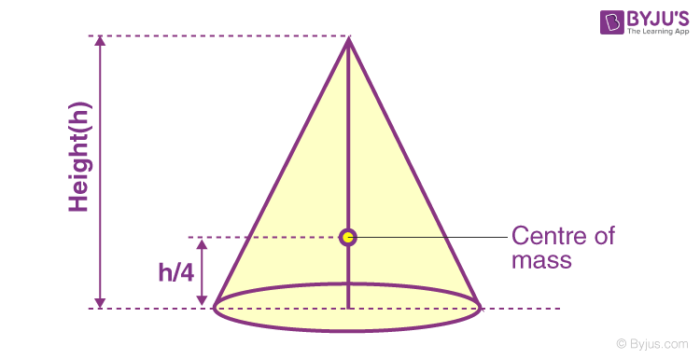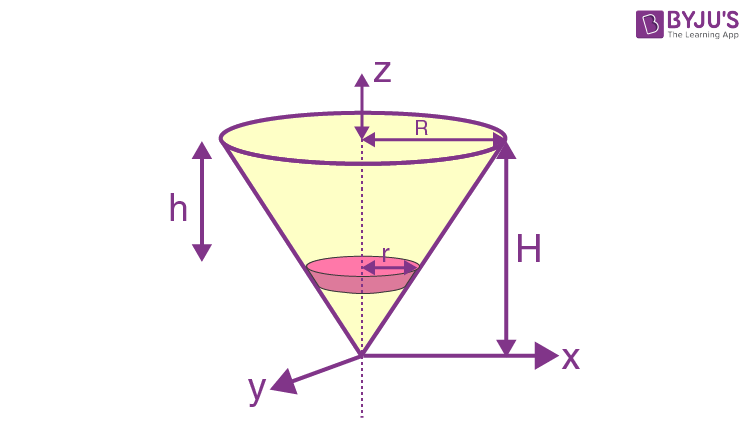Win up to 100% scholarship on Aakash BYJU'S JEE/NEET courses with ABNAT Win up to 100% scholarship on Aakash BYJU'S JEE/NEET courses with ABNAT

# Centre of Mass of a Solid Cone

Centre of Mass of a system or a body is a point where the whole of the mass of the system will be concentrated. This point-like mass has the same type of translational motion as the system as a whole if the net external force acting on the point mass is the same as that acting on the system.

The centre of mass (centre of gravity) of the cone is the point on the object about which the entire mass of the object is equally distributed. The cone is of two types: solid cone and hollow cone. Let us consider a solid cone kept on a horizontal surface with its apex in the air. Some reasonable observations can be made about the centre of mass.

Symmetry: The centre of mass will be along the line joining the apex to the centre of the base of the cone.

Shape: We can see that there is more mass at the lower half of the cone. Therefore, the centre of mass will be closer to the base than the apex.

The centre of mass of the solid cone will lie on the altitude at a distance of h/4 distance from the centre of the base of the cone.Centre of mass = h/4,

Where h is the height of the cone

## How to Calculate the Centre of Mass of the Solid ConeConsider the cone as in the above figure. Considering the symmetry of the cone we know that the centre of mass is in the line joining the centre of the base of the cone and the apex.

The equation of centre of mass

$$\begin{array}{l}C =\frac{\int \rho hdV}{\int \rho dV}\end{array}$$

Where ρ is the density of the material of the cone.

Let us consider a small slice of the cone of thickness dh and radius r at a height h from the base of the cone.

r/R = (H – h)/H ⇒ r = (H – h)R/H

The volume of the small slice is dV = πr2dh = π[(H – h)R/H]2dh

$$\begin{array}{l}C =\frac{\int_{0}^{H} \rho hdV}{\int_{0}^{H} \rho dV}\end{array}$$
$$\begin{array}{l}C =\frac{\int_{0}^{H} hdV}{\int_{0}^{H} dV}———(1)\end{array}$$

Finding the value of

$$\begin{array}{l}\int_{0}^{H}h dV = \int_{0}^{H}h\pi \left ( \frac{(H -h)R}{H} \right )^{2}dh = \frac{\pi R^{2}}{H^{2}}\int_{0}^{H}(H^{2}h-2Hh^{2}+h^{3})dh\end{array}$$

= πR2H/12 ———(2)

Finding the value of

$$\begin{array}{l}\int_{0}^{H} dV = \int_{0}^{H}\pi \left ( \frac{(H -h)R}{H} \right )^{2}dh = \frac{\pi R^{2}}{H^{2}}\int_{0}^{H}(H^{2}-2Hh+h^{2})dh\end{array}$$

= πR2/3 ———(3)

Substituting equa (2) and equa (3) in equa (1) we get

$$\begin{array}{l}C =\frac{\int_{0}^{H} hdV}{\int_{0}^{H} dV}\end{array}$$
= [πR2H/12]/ [πR2/3] = H/4

#### Centre of Mass of a Uniform Solid Cone## Frequently Asked Questions on Centre of Mass of a Solid Cone

Q1

### Where does the centre of mass of a solid cone lie?

The solid cone’s centre of mass will be 3/4 of the distance from the apex of the cone on the altitude.

Q2

### What are the two types of cones?

Solid cone and hollow cone.

Q3

### How to find the centre of mass of a solid cone?

The centre of mass of a solid cone can be determined by taking a suitable cross-section of the body, applying the centre of mass formula for continuous bodies and integrating it throughout.

Test Your Knowledge On Centre Of Mass Of A Solid Cone!